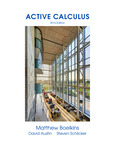Select to expand
Select to collapse

# Course ePortfolio

## Single Variable Calculus I Early Transcendentals

This collection contains the California Open Educational Resources Council (CA OER) list of peer-reviewed textbooks for Single Variable Calculus I Early Transcendentals (common course-id MATH 210), one of the top 50 college courses identified for inclusion in the California Open Online Library for Education (COOLforEd).

CID Number: MATH 210

#### General Course Description: MATH 210

A first course in differential and integral calculus of a single variable: functions; limits and continuity; techniques and applications of differentiation and integration; Fundamental Theorem of Calculus. Primarily for Science, Technology, Engineering & Math Majors.

Minimum Units: 4.0

Share

### Prerequisites

Prerequisites: Pre-calculus, or college algebra and trigonometry, or equivalent.

Corequisites: None

### Pedagogical Approach & Learning Outcomes

None

#### Learning Outcomes

Upon successful completion of the course, students will be able to:

• Compute the limit of a function at a real number;
• Determine if a function is continuous at a real number;
• Find the derivative of a function as a limit;
• Find the equation of a tangent line to a function;
• Compute derivatives using differentiation formulas;
• Use differentiation to solve applications such as related rate problems and optimization problems;
• Use implicit differentiation;
• Graph functions using methods of calculus;
• Evaluate a definite integral as a limit;
• Evaluate integrals using the Fundamental Theorem of Calculus; and
• Apply integration to find area.

### Assessment & Other Information

#### Assessment

• Tests,
• examinations,
• homework or
• projects where students demonstrate their mastery of the learning objectives and their ability to devise, organize and present complete solutions to problems.

None

### Course Resources

•Calculus - Early Transcendentals (Open (Access) Textbook)
The discussions and explanations are succinct and to the point, in a way that pleases mathematicians who do not like... More
•Calculus (Open (Access) Textbook)
Published in 1991 and still in print from Wellesley-Cambridge Press, the book is a useful resource for educators and... More
•Mooculus: Calculus (Open (Access) Textbook)
This open textbook is a first and friendly introduction to calculus, suitable for someone who has never seen the subject... More
•Active Calculus (Open (Access) Textbook)
While each author of a calculus textbook certainly offers her own creative perspective on the subject, it is hardly the... More